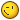# Found some unnecessary calculations

## Recommended Posts

Not sure if here's the best place for this but since it is not a bug etc I avoided opening an issue on githubI stumbled upon some odd rotation code in phaser while reading https://phaser.io/tutorials/advanced-rendering-tutorial/part7

From src/pixi/renderers/webgl/utils/WebGLSpriteBatch.js
and src/pixi/display/Sprite.js

``````// Rotate matrix by 90 degrees
// We use precalculated values for sine and cosine of rad(90)
a = a0 * 6.123233995736766e-17 + -c0;
b = b0 * 6.123233995736766e-17 + -d0;
c = a0 + c0 * 6.123233995736766e-17;
d = b0 + d0 * 6.123233995736766e-17;``````

Ok. Some rotationcode with fancy precalculated values.

But: the value given in e-notation is 0.00000000000000006123233995736766 and therefore effectively zero.
Which makes sense since this was meant to be the precalculation of cos(rad(90)) - which equals exactly to zero.
I suppose the literal resulted from a rounding error when someone typed Math.cos(Math.radians(90)) into a consoleSo a better version would be:

``````// Rotate matrix by 90 degrees
a = -c0;
b = -d0;
c = a0;
d = b0;``````

Maybe someone sees this and is willing to fix it on the side...